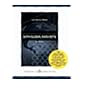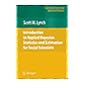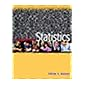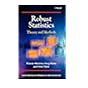Normal view

# Introduction to applied Bayesian statistics and estimation for social scientists / Scott M. Lynch.

Material type:TextLanguage: English Publication details: c2007. Description: xxviii, 357 p. : ill. ; 24 cmISBN: 9780387712642 (hardcover : alk. paper)DDC classification: 519.5 LYI LOC classification: HA29 | .L973 2007Online resources: Table of contents | OCLC | E-book Fulltext
Contents:
1. Introduction -- 2. Probability theory and classical statistics -- 3. Basics of Bayesian statistics -- 4. Modern model estimation part 1 : Gibbs sampling -- 5. Modern model estimation part 2 : Metropolis-Hastings sampling -- 6. Evaluating Markov chain Monte Carlo algorithms and model fit -- 7. The linear regression model -- 8. Generalized linear models -- 9. Introduction to hierarchical models -- 10. Introduction to multivariate regression models -- 11. Conclusion -- A. Background mathematics -- B. The central limit theorem, confidence intervals, and hypothesis tests.
Summary: Lynch covers the complete process of Bayesian statistical analysis in great detail from the development of a model through the process of making statistical inference. The key feature of the book is that it covers models that are most commonly used on social science research.
Tags from this library: No tags from this library for this title.
Star ratingsAverage rating: 0.0 (0 votes)
Holdings
Item type Current library Collection Call number Copy number Status Date due Barcode Item holdsE-Book
E-book
Non-fiction 519.5 LYI 2007 (Browse shelf(Opens below)) Not For LoanText
Reserve Section
Non-fiction 519.5 LYI 2007 (Browse shelf(Opens below)) C-1 Not For Loan 25614Text
Circulation Section
Non-fiction 519.5 LYI 2007 (Browse shelf(Opens below)) C-2 Available 26049
Total holds: 0
##### Browsing EWU Library shelves, Shelving location: Reserve Section Close shelf browser (Hides shelf browser)519.5 LIS 2008 Statistical techniques in business & economics with global data sets. 519.5 LIS 2012 Statistical techniques in business & economics / 519.5 LIS 2015 Statistical techniques in business and economics / 519.5 LYI 2007 Introduction to applied Bayesian statistics and estimation for social scientists / 519.5 MAI 2004 Introductory statistics / 519.5 MAR 2006 Robust statistics : 519.5 MAS 1981 Statistics :

Includes bibliographical references (p. -351) and index.

1. Introduction --
2. Probability theory and classical statistics --
3. Basics of Bayesian statistics --
4. Modern model estimation part 1 : Gibbs sampling --
5. Modern model estimation part 2 : Metropolis-Hastings sampling --
6. Evaluating Markov chain Monte Carlo algorithms and model fit --
7. The linear regression model --
8. Generalized linear models --
9. Introduction to hierarchical models --
10. Introduction to multivariate regression models --
11. Conclusion --
A. Background mathematics --
B. The central limit theorem, confidence intervals, and hypothesis tests.

Lynch covers the complete process of Bayesian statistical analysis in great detail from the development of a model through the process of making statistical inference. The key feature of the book is that it covers models that are most commonly used on social science research.

AS

Tahur Ahmed

There are no comments on this title.